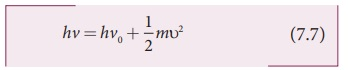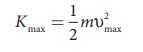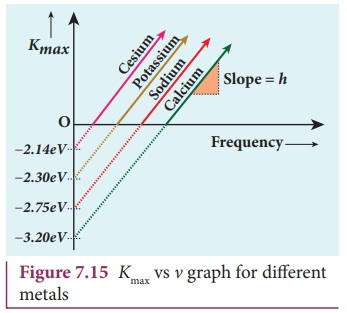Home | | Physics 12th Std | Particle nature of light: EinsteinŌĆÖs explanation

# Particle nature of light: EinsteinŌĆÖs explanation

According to Einstein, the energy in light is not spread out over wavefronts but is concentrated in small packets or energy quanta.

PHOTO ELECTRIC EFFECT

Particle nature of light: EinsteinŌĆÖs explanation

Einstein extended PlanckŌĆÖs quantum concept to explain the photoelectric effect in 1905. According to Einstein, the energy in light is not spread out over wavefronts but is concentrated in small packets or energy quanta. Therefore, light (or any other electromagnetic waves) of frequency v from any source can be considered as a stream of quanta and the energy of each light quantum is given by E = h╬Į.

He also proposed that a quantum of light has linear momentum and the magnitude of that linear momentum is p = h╬Į / c . The individual light quantum of definite energy and momentum can be associated with a particle. The light quantum can behave as a particle and this is called photon. Therefore, photon is nothing but particle manifestation of light.

## Characteristics of photons:

According to particle nature of light, photons are the basic constituents of any radiation and possess the following characteristic properties:

i) The photons of light of frequency ╬Į and wavelength ╬╗ will have energy, given by E = hv = hc/╬╗ .ii) The energy of a photon is determined by the frequency of the radiation and not by its intensity and the intensity has no relation with the energy of the individual photons in the beam.

iii) The photons travel with the velocity of light and its momentum is given by p = h/╬╗ = hv/civ) Since photons are electrically neutral, they are unaffected by electric and magnetic fields.

v) When a photon interacts with matter (photon-electron collision), the total energy, total linear momentum and angular momentum are conserved. Since photon may be absorbed or a new photon may be produced in such interactions, the number of photons may not be conserved.

According to quantum concept, intensity of light of given wavelength is defined as the number of energy quanta or photons incident per unit area per unit time, with each photon having same energy. The unit is WmŌĆō2.

## EinsteinŌĆÖs explanation of photoelectric equationWhen a photon of energy h╬Į is incident on a metal surface, it is completely absorbed by a single electron and the electron is ejected. In this process, a part of the photon energy is used for the ejection of the electrons from the metal surface (photoelectric work function ŽĢ0 ) and the remaining energy as the kinetic energy of the ejected electron. From the law of conservation of energy,where m is the mass of the electron and Žģ its velocity. This is shown in Figure 7.13(a).If we reduce the frequency of the incident light, the speed or kinetic energy of photo electrons is also reduced. At some frequency ╬Į0 of incident radiation, the photo electrons are ejected with almost zero kinetic energy (Figure 7.13(b)). Then the equation (7.6) becomes

hv0 = ŽĢ0

where ╬Į0 is the threshold frequency. By rewriting the equation (7.6), we getThe equation (7.7) is known as EinsteinŌĆÖs photoelectric equation.

If the electron does not lose energy by internal collisions, then it is emitted with maximum kinetic energy Kmax. Thenwhere Žģmax is the maximum velocity of the electron ejected. The equation (7.6) is rearranged as follows:A graph between maximum kinetic energy Kmax of the photoelectron and frequency ╬Į of the incident light is a straight line as shown in Figure 7.14. The slope of the line is h and its y-intercept is ŌĆōŽĢ0.

EinsteinŌĆÖs equation was experimentally verified by R.A. Millikan. He drew Kmax versus ╬Į graph for many metals (cesium, potassium, sodium and lithium) as shown in Figure 7.15 and found that the slope is independent of the metals.Millikan also calculated the value of PlanckŌĆÖs constant (h=6.626├Ś10ŌĆō34 Js) and work function of many metals (Cs, K, Na, Ca); these values are in agreement with the theoretical prediction.

## Explanation for the photoelectric effect:

The experimentally observed facts of photoelectric effect can be explained with the help of EinsteinŌĆÖs photoelectric equation.

i) As each incident photon liberates one electron, then the increase of intensity of the light (the number of photons per unit area per unit time) increases the number of electrons emitted thereby increasing the photocurrent. The same has been experimentally observed.

ii) From Kmax = hv ŌĆō ŽĢ0, it is evident that Kmax is proportional to the frequency of the light and is independent of intensity of the light.

iii) As given in equation (7.7), there must be minimum energy (equal to the work function of the metal) for incident photons to liberate electrons from the metal surface. Below which, emission of electrons is not possible. Correspondingly, there exists minimum frequency called threshold frequency below which there is no photoelectric emission.

iv) According to quantum concept, the transfer of photon energy to the electrons is instantaneous so that there is no time lag between incidence of photons and ejection of electrons.

Thus, the photoelectric effect is explained on the basis of quantum concept of light.

## The nature of light: wave - particle duality

We have learnt that wave nature of light explains phenomena such as interference, diffraction and polarization. Certain phenomena like black body radiation, photoelectric effect can be explained by assigning particle nature to light. Therefore, both theories have enough experimental evidences.

In the past, many scientific theories have been either revised or discarded when they contradicted with new experimental results.

Here, two different theories are needed to answer the question: what is nature of light?

It is therefore concluded that light possesses dual nature, that of both particle and wave. It behaves like a wave at some circumstances and it behaves like a particle at some other circumstances.

In other words, light behaves as a wave during its propagation and behaves as a particle during its interaction with matter. Both theories are necessary for complete description of physical phenomena. Hence, the wave nature and quantum nature complement each other.

A reader may find it difficult to understand how light can be both a wave and a stream of particle. This is the case even for great scientist like Albert Einstein.

Einstein once wrote a letter to his friend Michel Besso in 1954 expressing his frustration:

ŌĆ£All these fifty years of conscious brooding have brought me no closer to answer the question, ŌĆśWhat are light quanta?ŌĆÖ Of course today everyone thinks he knows the answer, but he is deluding himself ŌĆØ.

Tags : Photo Electric Effect | Physics , 12th Physics : UNIT 8 : Dual Nature of Radiation and Matter
Study Material, Lecturing Notes, Assignment, Reference, Wiki description explanation, brief detail
12th Physics : UNIT 8 : Dual Nature of Radiation and Matter : Particle nature of light: EinsteinŌĆÖs explanation | Photo Electric Effect | Physics